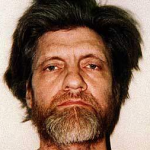The Unabomber (Ted Kaczynski) is a serial killer. Between 1978 and 1995, he engaged in a nationwide bombing campaign, mailing or planting self-made bombs, killing three people and injuring 23 others. After his arrest in 1996 the police found encrypted notes in his possession. These notes have never been published, but some fragments have leaked. This page aims to gather all the information publicly known about the encrypted notes of the Unabomber.

## Encrypted Notes

Encrypted document created by the Unabomber (sold at an auction):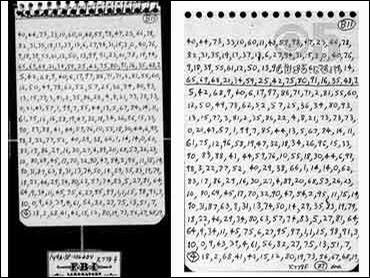The same document (sold at an auction, source):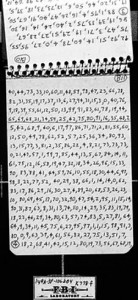The same document (scan from this video):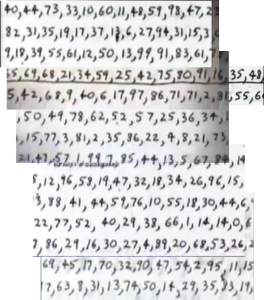Encrypted note (source):Encrypted note sold at an auction (source):Encrypted note (source, transcription):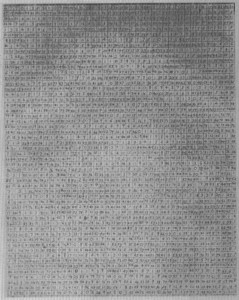## Key Material

The police cracked the Unabomber’s code using key material found in his possession. This is a substitution table (there is no higher resolution available):This is another substitution table: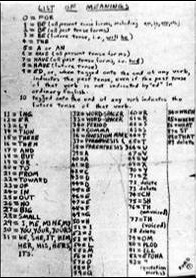And yet another one: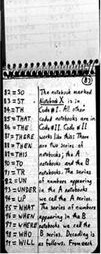And yer another one (source):This document indicates the order in wich the letters are to be read:Substitution table (source):
0=for
1=be (all present tense forms, including am, is, are, etc.)
2=be (all past tense forms)
3=be(all future tense; i.e., will b)
4=the
5=a or an
6=have (all present tense forms)
7=have (all past tense forms; i.e. had)
8=have (future tense)
9=ed, or, when tagged onto the end of any verb, indicates the past tense, even if the past tense of that verb is not indicated by “ed” in ordinary English.
10=tagged onto theend of any verb indicates the future tense of that verb.
11=ing
50=H
12=er
51=I
13=ly
52=J
14=tion
53=K
15=there
54=L
16=then
55=M
17=and
56=N
18=but
57=O
19=or
58=P
20=to
59=Q
21=from
60=R
22=toward
61=R
23=at
62=S
24=in
63=S
25=out
64=T
26=no
65=T
27=big
66=U
28=small
67=V
29=I, me , mine, my
68=W
30=you, your, yours
69=X
31=he, she, it, him, her, his, hers, its
70=Y
32=word spacer
71=Z
33=word spacer
72=delete
34=period
73=delete
35=comma
74=ch
36=question mark
75=sh
37=parenthesis (
76=th (numerical)
38=parenthesis )
77=th (voiced)
39=A
78=delete
40=A
79=OM
41=B
80=PLOD
42=C
81=ILL
43=D
82=ETONA
44=D
83=“ (quotation marks)
45=E
84=when
46=E
85=where
47=E
86=what
48=F
87=st
49=G
88=that
89=delete
Here is another substitution table (taken from the video below, transcribed by Nick Pelling):
82 = SO
83 = ST
84 = TH
85 = THAT
86 = THE
87 = THERE
88 = THEN
89 = THIS
90 = TO
91 = TR
92 = UN
93 = UNDER
94 = UP
95 = WHAT
96 = WHEN
97 = WHERE
98 = WHO
99 = WH[Y?]

tbd.

## Solutions

One cleartext reads as follows (taken from the video referenced below): I sent a bomb to a computer expert … revenge attempts have been gobbling much time, impeeding other work. But I must succeed, must get revenge.

## Literature

There is a lot of literature about the Unabomber, but most of it doesn’t cover his encrypted notes in detail. The following sources are the best I know:

## Kommentare (1)

1. #1 David Oranchakhttp://zodiackillerciphers.com
3. Februar 2023